Courses

# Electronic Devices & Digital Communications - MCQ Test - 1

## 15 Questions MCQ Test GATE ECE (Electronics) 2022 Mock Test Series | Electronic Devices & Digital Communications - MCQ Test - 1

Description
This mock test of Electronic Devices & Digital Communications - MCQ Test - 1 for Electronics and Communication Engineering (ECE) helps you for every Electronics and Communication Engineering (ECE) entrance exam. This contains 15 Multiple Choice Questions for Electronics and Communication Engineering (ECE) Electronic Devices & Digital Communications - MCQ Test - 1 (mcq) to study with solutions a complete question bank. The solved questions answers in this Electronic Devices & Digital Communications - MCQ Test - 1 quiz give you a good mix of easy questions and tough questions. Electronics and Communication Engineering (ECE) students definitely take this Electronic Devices & Digital Communications - MCQ Test - 1 exercise for a better result in the exam. You can find other Electronic Devices & Digital Communications - MCQ Test - 1 extra questions, long questions & short questions for Electronics and Communication Engineering (ECE) on EduRev as well by searching above.
QUESTION: 1

### Concentration of free electrons in a copper wire (diameter=1.03 mm) is 8.4 x 1028 electrons/m3. If the current in the wire is 2A, then drift velocity is

Solution: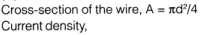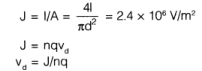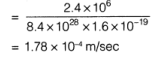QUESTION: 2

### If a donor-type impurity is added to the extent of 1 atom per 108 silicon atoms, find the resistivity.

Solution: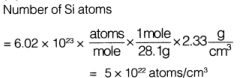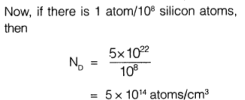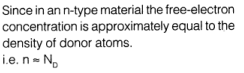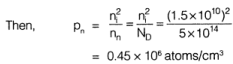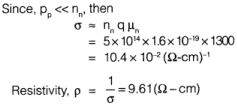QUESTION: 3

### The p-type substrate in a monolithic circuit should be connected to

Solution:
QUESTION: 4

What is the ratio of current for a forward bias of 0.05 V to the current for the same magnitude of reverse bias?

Solution: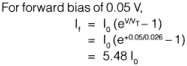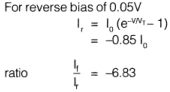QUESTION: 5

The probability of an electron being thermally promoted to the conduction band in Si(∈g=1.107 eV) at room temperature (25°C)?

Solution:
QUESTION: 6

One curve of an n-channel MOSFET is characterized by the following parameters
ID(sat) = 0.2 mA
VDs = 0.8 V

VTN =0.8V

The value of conduction parameter is

Solution: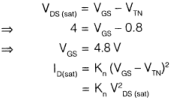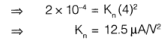QUESTION: 7

Sampling rate needed to achieve (SNR)0/P ≥ 45 dB with fs = 10 MHz in a PCM system

Solution: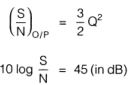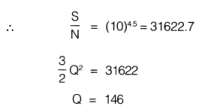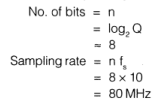QUESTION: 8

In a 64 step binary PCM system with a reference scale from 0 to 6.4 volts, the RMS quantization error will be,

Solution: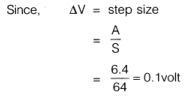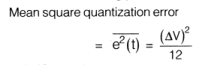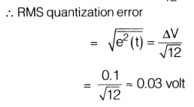QUESTION: 9

In a BPSK signal detector, the local oscillator has a fixed phase error of 25.2°. Reduction in SNR will be by a factor of _____________

Solution: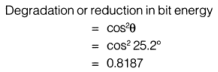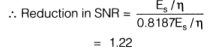QUESTION: 10

The SQR (Signal to Quantization Noise Ratio) of a system is 25.8 dB for 16 quantization levels. The percentage increase in SQR for 128 quantization levels is

Solution: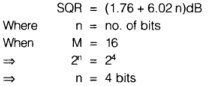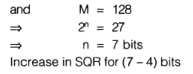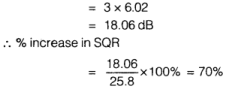QUESTION: 11

Error distance in 8 QAM modulation scheme is

Solution: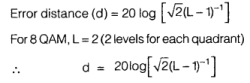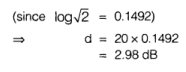QUESTION: 12

A system with transfer function H(s) is driven by a white noise x(t) with power density N0. The Auto-correlation of the output signal is measured as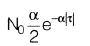where α > 0. A possible transfer function H(s) for system wilt be

Solution: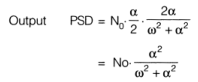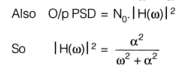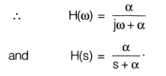QUESTION: 13

A vestigial SSB system is used to modulate a TV signal having bandwidth equal to 4 MHz. If roll-off factor is 1, the bandwidth required to transmit the signal is given by _________ MHz

Solution:
QUESTION: 14

The capacitance of varactor varies from 5 to 50 pF. Two such varactor diodes are used in the tunning circuit shown in figure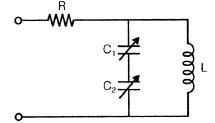If L= 10 mH, the tuning range of the circuit

Solution: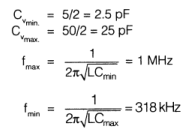QUESTION: 15

The variation of drain current with gate to source voltage (lD - VGS characteristic) of a MOSFET is shown in figure. The MOSFET is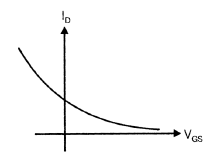Solution: